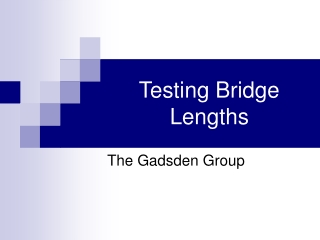DownloadDownload PresentationTesting Bridge Lengths

# Testing Bridge Lengths

Télécharger la présentation## Testing Bridge Lengths

- - - - - - - - - - - - - - - - - - - - - - - - - - - E N D - - - - - - - - - - - - - - - - - - - - - - - - - - -
##### Presentation Transcript

1. Testing Bridge Lengths The Gadsden Group

2. Goals and Objectives • Collect and express data in the form of tables and graphs • Look for patterns to make predictions from tables and graphs • Distinguish between linear and non-linear relationships from tables and graphs

3. Standards and Benchmarks • Standard #2: Algebra • Definition: Students will understand algebraic concepts and applications. • Benchmark #2-D: • Analyze changes in various contexts • 1. Use graphs, tables, and algebraic representations to make predictions and solve problems that involve change. • 2. Estimate, find, and justify solutions to problems that involve change using tables, graphs, and algebraic expressions. • 3. Use appropriate problem-solving strategies (e.g., drawing a picture, looking for a pattern, systematic guessing and checking, acting it out, making a table or graph, working a simpler problem, writing an algebraic expression or working backward) to solve problems that involve change. • 5. Analyze problems that involve change by identifying relationships, distinguishing relevant from irrelevant information, identifying missing information, sequencing, and observing patterns. • 7. Recognize the same general pattern of change presented in different representations.

4. Standards and Benchmarks cont’d • Standard #5: Data Analysis and Probability • Definition: Students will understand how to formulate questions, analyze data, and determine probabilities. • Benchmark #5-A: • 1. Represent two numerical variables on a plot, describe how the data points are distributed and identify relationships that exist between the two variables. • 3. Organize, analyze, and display appropriate quantitative and qualitative data to address specific questions including: plots charts and tables. • 5. Simulate an event selecting and using different models. • Benchmark #5-B: • 3. Analyze data to make decisions and to develop convincing arguments from data displayed in a variety of formats that include: graphs scatter plots charts and tables • 4. Interpret and analyze data from graphical representations and draw simple conclusions (e.g., line of best fit). • 5. Evaluate and defend the reasonableness of conclusions drawn from data analysis. • 7. Identify simple graphic misrepresentations and distortions of sets of data (e.g., unequal interval sizes, omission of parts of axis range, scaling).

5. Standards and Benchmarks cont’d • Benchmark #5-C: • Develop and evaluate inferences and predictions that are based on data • 2. Describe how reader bias, measurement errors, and display distortion can affect the interpretation of data, predictions, and inferences based on data. • 3. Conduct simple experiments and/or simulations, record results in charts, tables, or graphs, and use the results to draw conclusions and make predictions. • 4. Compare expected results with experimental results and information used in predictions and inferences.

6. Lesson: Non-Linear Models “In this investigation, students will encounter a different kind of relationship: an inverse relationship. In an inverse relationship, as one variable decreases the other increases, but not in a linear fashion - that is, not by constant decreases. There is an underlying constant in inverse relationships: when the two variables are multiplied, they yield a constant product. Students will see that the pattern of change in the data is similar to that in the other inverse relationships they will investigate.” (Lappan, et al, Thinking With Mathematical Models, p. 36a).

7. Lesson Cont’d • Students will explore non-linear relationships by testing the lengths of bridges and their breaking weight. • Launch: • What do you expect to happen in this experiment? • We are using equipment similar to what we have used before. What are the variables this time? (Length and breaking weight) • What will the data look like? What shape do you think the graph will be?

8. Lesson cont’d • Explore: • Have students conduct the experiment and discuss the questions within their group. • Ask each student to make a table/graph and write his or her own answers. • Each group will compare their data and come to a consensus in order to create a group poster of their table and graph.

9. Lesson cont’d • Summarize: • After students have displayed and presented their group work, then ask: • Are there similarities in the results of the various groups? Are there differences? What might have caused those differences? • As bridge length increases, what happens to the number of pennies that can be supported? (It decreases) • As bridge length decreases, what happens to the number of pennies that can be supported? (It increases) • Focus the class’s attention on this inverse relationship. • What shape or pattern do you see in your graph? Is it linear? (no, it is definitely a curve) • How might you be able to tell from your table that the graph model might be a curve? (The difference between two consecutive breaking weights decreases less and less as the length increases; it is not a constant change)